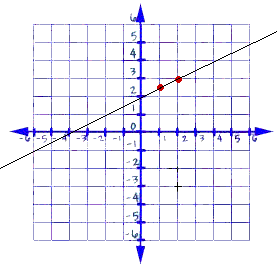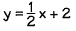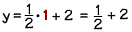In this lesson, we have summarized the basic concepts and functionalities of Linear Equations with Two Variables Class 9. Let us look at the definitions and rules involved in solving a linear equation.

## What is a Linear Equation?

Linear equations are those set of equations that have both real numbers and variables. For example, if we consider the equation ax + by + c = 0;

a, b and c are real numbers

x and y are termed as variables.

In the above equation, a is termed as the coefficient of x, b is the coefficient of y and c is known as the constant term.

Here are some examples of a linear equation in two variables:

6x  + 4y + 7 = 0

3x + 2y +11 = 0

## What is the solution of a Linear Equation?

The value of any variable which is to be substituted for the variable in the equation; when it satisfies the equation, which is to say that the left-hand side is identical to the right-hand side of the equation; this is known as the solution of a linear equation.

It is also commonly known as the root of a linear equation.

## What are the rules for solving a Linear Equation?

1. It is possible to add the same quantity to both sides of the equation while not changing the equality.
2. It is permissible to subtract the same quantity to both sides of the equation without changing the equality.
3. The two sides of the equation can be multiplied by a non zero entity or number while not changing the equality.
4. Both sides of the equation may be divided by a non zero number while not changing the equality.

## How many solutions does a Linear Equation in two variables have?

The answer to the above question is infinite. Yes, you read that right. Read the following paragraph to understand the concept better.

Consider the following equation:

2x + 3y = 12

Pick a value of your choice for the variable x. Let us say, for example, that you have chosen x=2.

In this case, this linear equation in tow variables reduces to a linear equation in one variable because when we substitute x=2 the equation turns into 4 + 3y = 12.

So on solving the above equation, you get the value of y, which is y= 8/3

So in this scenario, you get two solutions: x = 2 and y = 8/3

If you consider another case where the value you have given to x is x = -5, you will realize that the equation change to -10 + 3y = 12.

On further simplifying the equation, you get 3y = 22 and similarly y = 22/3.

So in this case, you have another set of solutions where x = -5 and y = 22/3

As a result, there is n end to the number of solution for a linear equation in two variables. Hence it is said that a linear equation in two variables has an infinite number of solutions as stated in the above paragraph.

However, an easier way of obtaining the solution of a linear equation in two variables is by making the value of x zero. Then we can get the corresponding value of y. in the same way, we can put the value of y zero to get the equivalent value of x.

## Graph of Linear Equations

The set of all the points on the graph that coordinate perfectly with the equation is the graph of the equation in x and y.

with the intention of  drawing a graph for the linear equation of two variables ax + by + c = 0, the following steps can be used:

1. Get the linear equation ax + by + c = 0
2. Express y in terms of x. that is y = -((ax + b)/c))
3. Put any two to three values for x and get the corresponding values for y. therefore you will get three sets of points to mark on the graph.
4. Plot the points on the graph paper.
5. Join the points on the graph paper. The line hence obtained is the graph of the linear equation in two variables ax + by +c = 0.

Please note, the reason that a degree one polynomial equation ax  + by + c = 0 is termed as a linear equation is it is a geometrical representation in a straight line.

Also, the graph of the equation y = kx is a line which always passes through the origin of the graph.

## Equations of Lines parallel to the X-axis and the Y-axis

It is common knowledge that every point on the x-axis is  (x,0). The equation for the x-axis is given by y = 0.

Similarly, the equation for the y-axis can be figured out by x = 0.

The following diagram indicates an example of a linear equation where the solution is obtained and then plotted on a graph.From the above graph, we can deduce that the solution of this equationis.

These were the basic concepts of Linear Equations in Two Variables. To know more about the various methods required to solve linear equations, visit here!

+91
No thanks.

## Request a Free 60 minute counselling session at your home

Please enter a valid phone number
•7,829,648

Happy Students
•358,177,393

Questions Attempted
•3,028,498

Tests Taken
•3,020,367